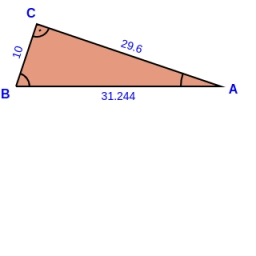# Perpendicular 41811

Calculate the area of a right triangle whose longer perpendicular is six dm shorter than the hypotenuse and three dm longer than the shorter perpendicular.

S =  158.9423 dm2

### Step-by-step explanation:Did you find an error or inaccuracy? Feel free to write us. Thank you!

Tips for related online calculators
Are you looking for help with calculating roots of a quadratic equation?
Do you have a linear equation or system of equations and looking for its solution? Or do you have a quadratic equation?